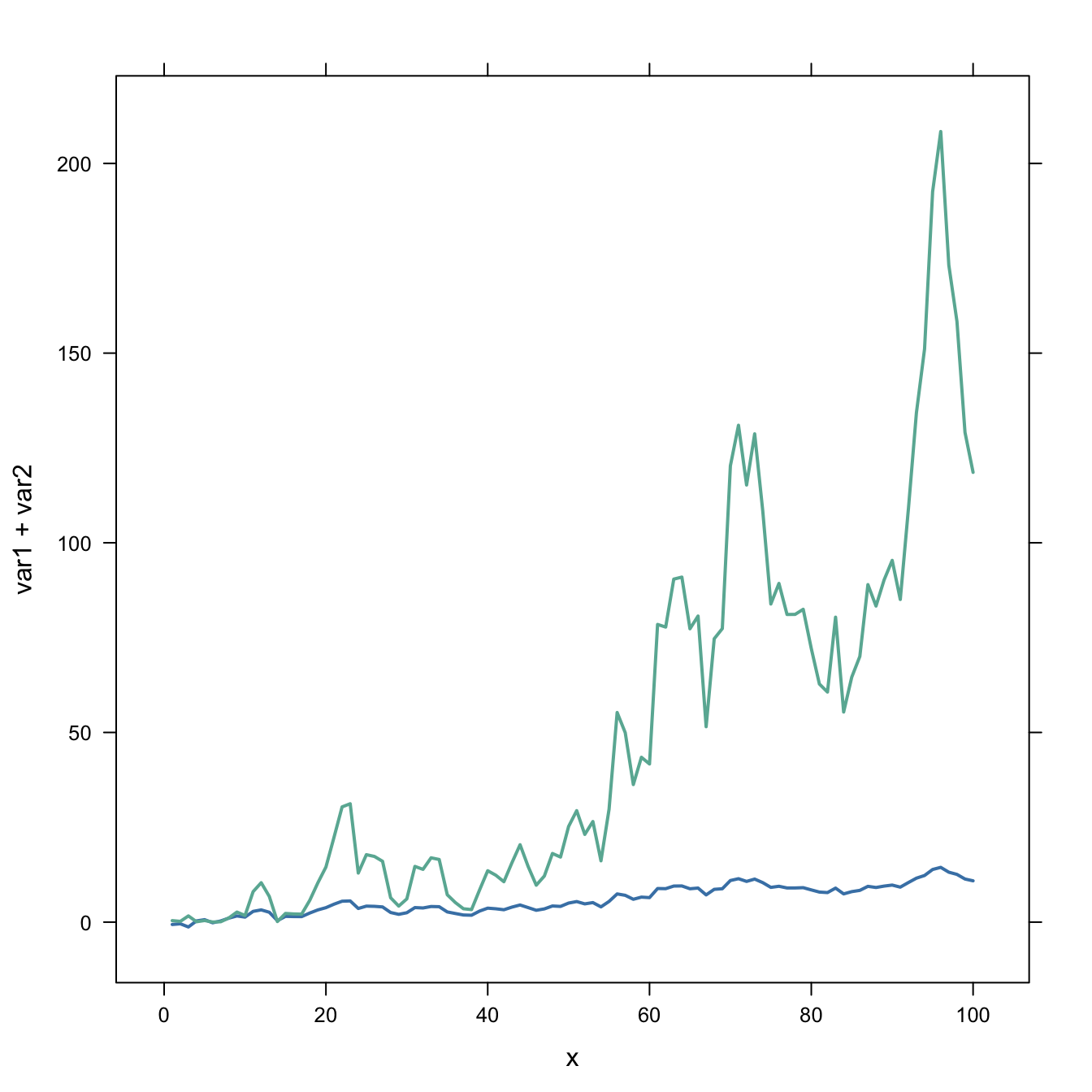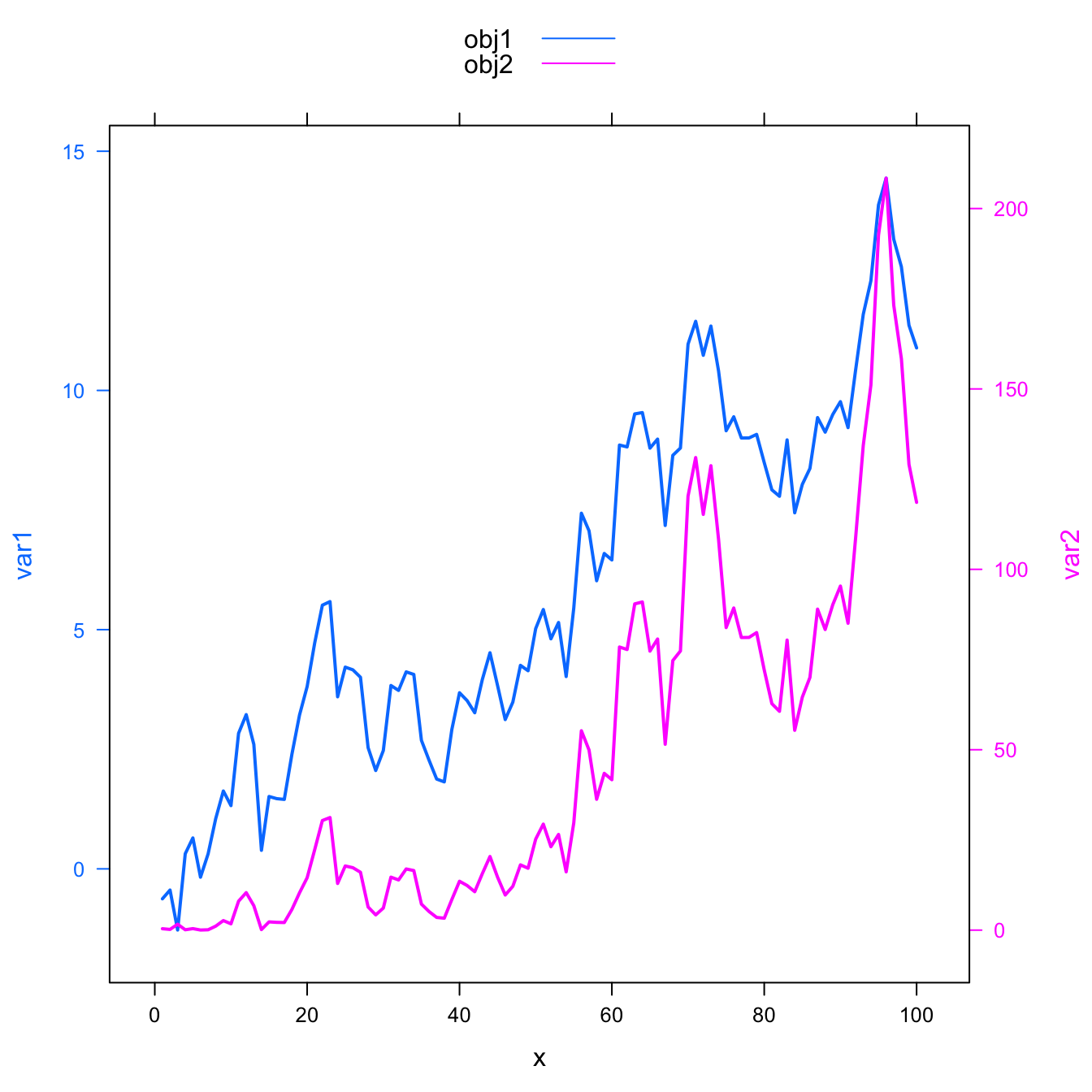# Dual Y axis in R

This post explains how to build a line chart with dual Y axis with R. It show the evolution of 2 series, each line having its own Y axis. Reproducible code provided.

# Warning

Warning: a dual Y axis line chart represents the evolution of 2 series, each plotted according to its own Y scale. This kind of chart must be avoided, since playing with Y axis limits can lead to completely different conclusions. Visit data-to-viz for more info.

# Usual line chart

Let’s start with an usual line chart displaying the evolution of 2 numeric variables.

This kind of chart can be built using the `line()` function. But here the `xyplot` from the `latticeExtra` package is used (we’ll need it later on.)

One would argue that the exact evolution of the blue variable is hard to read. Another axis could be displayed to zoom on it. This is why the dual axis was born.``````#library
library(latticeExtra)

# create data
set.seed(1)
x <- 1:100
var1 <- cumsum(rnorm(100))
var2 <- var1^2
data <- data.frame(x,var1,var2)

# usual line chart
xyplot(var1 + var2 ~ x, data, type = "l", col=c("steelblue", "#69b3a2") , lwd=2)``````

# Dual Y axis line chart

The `doubleYScale()` function of the `latticeExtra` package can take 2 outputs of the `xyplot()` function to build a dual Y axis line chart.

This chart is truly misleading: it is easy to conclude that both variables follow the same pattern what is totally wrong. Always double check what axis trully are on dual axis chart.

Conclusion: don’t do it!``````#library
library(latticeExtra)

# create data
set.seed(1)
x <- 1:100
var1 <- cumsum(rnorm(100))
var2 <- var1^2
data <- data.frame(x,var1,var2)

# --> construct separate plots for each series
obj1 <- xyplot(var1 ~ x, data, type = "l" , lwd=2, col="steelblue")
obj2 <- xyplot(var2 ~ x, data, type = "l", lwd=2, col="#69b3a2")

# --> Make the plot with second y axis:
doubleYScale(obj1, obj2, add.ylab2 = TRUE, use.style=FALSE )``````

If you stick to it, here is how to add a legend to the chart:``````#library
library(latticeExtra)

# create data
set.seed(1)
x <- 1:100
var1 <- cumsum(rnorm(100))
var2 <- var1^2
data <- data.frame(x,var1,var2)

# --> construct separate plots for each series
obj1 <- xyplot(var1 ~ x, data, type = "l" , lwd=2)
obj2 <- xyplot(var2 ~ x, data, type = "l", lwd=2)

# --> Make the plot with second y axis AND legend:
doubleYScale(obj1, obj2, text = c("obj1", "obj2") , add.ylab2 = TRUE)``````

Related chart types

## Contact

This document is a work by Yan Holtz. Any feedback is highly encouraged. You can fill an issue on Github, drop me a message on Twitter, or send an email pasting yan.holtz.data with gmail.com.